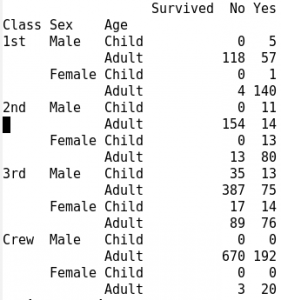Want to share your content on R-bloggers? click here if you have a blog, or here if you don't.The `ftable()` function combines Cross-Tabulation with the ability to format , or “flatten”, contingency tables of 3 or more dimensions.

The resulting tables contain the combined counts of the categorical variables, (also factor variables in R), that are then arranged as a matrix, whose rows and columns correspond to the original data’s rows and columns. The `ftable()` parameters, `row.vars` and `col.vars`, control the format of the table.

Displaying a contingency table in this flat matrix form is often preferable to showing it as a higher-dimensional array. The `write.ftable`, and `read.ftable`, functions allow for the saving, and accessing of Contigency Tables of 3 or more dimensions.

Answers to the exercises are available here.

Exercise 1
In order to demonstrate the `ftable()` function’s capabilities, input the `Titanic` data from R:

`data(Titanic)`

For the first exercise, create a basic flat contingency table from the Titanic data, using the `ftable()` function.

Exercise 2
The `row.vars` argument specifies the table variables that will format as table rows. `row.vars=` is definable with variable numbers, or the variable names.

Therefore, use `row.vars`= to specify the variable, `Class`, as the row variables.

Exercise 3
Combine the `row.vars` and `col.vars` arguments to specify `Class` & `Sex` as the row variables, and `Survived` as the column variable.

`row.vars` and `col.vars` are definable with variable numbers, or the variable names.

Exercise 4
With the parameters from the ftable used in Exercise 3, reverse the order of the `Class` and `Sex` columns.

Exercise 5
Next, using the `ftable()` code from Exercise 4, specify `Age` as the column variable.

As you can see from this exercise, `ftable()` allows for the formatting of data for different areas of inquiry.

Exercise 6
Using the `data.frame()` function will coerce ftable columns into rows. To demonstrate this, place the `ftable()` from Exercise 5, within the `data.frame()` function.

Exercise 7
Function `ftable.formula` provides a formula interface, (a data = . argument), for creating flat contingency tables.

For example:
`ftable(Survived ~ ., data = Titanic)`

Use the formula interface for `ftable()` to display the quantities in the `Titanic` data for Male/Female passengers, by `Class` and `Age`.

Exercise 8
The `ftable()` function creates an object of class `ftable`. In order to demonstrate this, save the results of the ftable formula from Exercise 5 as an ftable variable called `titanicStats`.

Exercise 9
Using the `write.table()` function, write the ftable, `titanicStats`, to a file. Make sure your working directory is set to a folder where you can find the resulting file. Name the file, “table1”.

Exercise 10
`read.ftable()` reads in a flat-like contingency table from a file.

Using `read.ftable()`, read the file, “table1”, into an R language environment variable called `data1`.